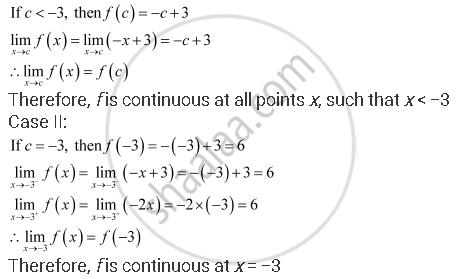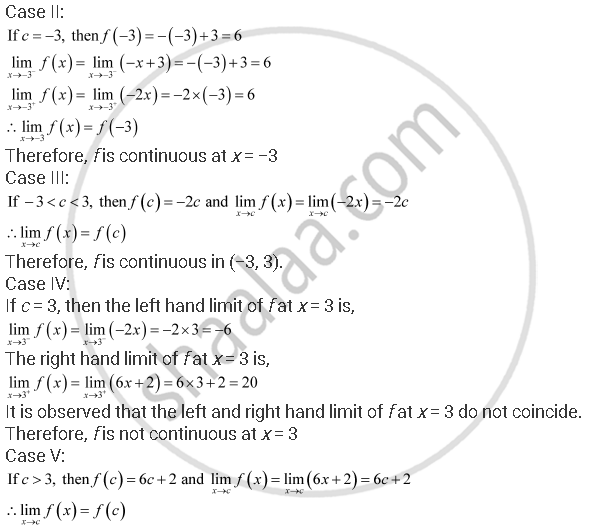Share

# Find All Points of Discontinuity Of F, Where F Is Defined by |X|+3, If X<= -3, -2x, If -3 < X < 3,6x + 2, If X >= 3 - Mathematics

#### Question

Find all points of discontinuity of f, where f is defined by f(x) = {(|x|+3, if x<= -3),(-2x, if -3 < x < 3),(6x + 2, if x >= 3):}

#### Solution

The given function f is f(x) = f(x) = {(|x|+3, if x<= -3),(-2x, if -3 < x < 3),(6x + 2, if x >= 3):}

The given function f is defined at all the points of the real line.

Let c be a point on the real line.

Case I:Therefore, f is continuous at all points x, such that x > 3

Hence, x = 3 is the only point of discontinuity of f.

Is there an error in this question or solution?